# NUMPY## Introduction to NumPy

#### Overview of the Library and Its Benefits

• NumPy, short for Numerical Python, is a popular library for data analysis and scientific computing in Python.
• It provides powerful tools for working with arrays, which are essential data structures for scientific computing.
• NumPy arrays are similar to lists in Python, but they offer several advantages for numerical computation.
• NumPy arrays are faster and more memory-efficient than lists, and they support vectorized operations, which enable you to perform computations on entire arrays at once.

#### Benefits:

• Efficient data handling: NumPy is designed to handle large multidimensional arrays and matrices efficiently, allowing for fast and efficient data manipulation and analysis.
• Powerful mathematical functions: NumPy provides a wide range of powerful mathematical functions, including linear algebra, Fourier transforms, and random number generation.
• Interoperability with other libraries: NumPy is designed to work seamlessly with other scientific computing libraries in Python, including Matplotlib, Pandas, and SciPy.
• Open-source: NumPy is open-source and freely available, allowing for widespread adoption and development by the scientific computing community.

#### Usage of Numpy?

NumPy arrays are the foundation of the library and are homogeneous, meaning that they contain elements of the same data type.

NumPy arrays can be created using various methods, such as by converting a list or tuple to an array or by using NumPy functions like linspace or arrange.

One of the main advantages of using NumPy is its speed and efficiency. NumPy arrays are much faster than Python lists and can handle large amounts of data efficiently.

This makes it an ideal choice for data processing tasks that require complex mathematical operations.

Installation

pip install numpy

NumPy can be installed using pip, a package management system in Python. Open your terminal or command prompt and run the following command:

#### Why do we import NumPy?

Working with NumPy entails importing the NumPy module before you start writing the code.

When we import NumPy as np, we establish a link with NumPy. We are also shortening the word “numpy” to “np” to make our code easier to read and help avoid namespace issues.

import numpy as np

The standard NumPy import, under the alias np, can also be named anything you want it to be.

Creating NumPy Arrays:

To create a NumPy array, you can use the numpy.array() function. This function takes a Python list as input and returns a NumPy array.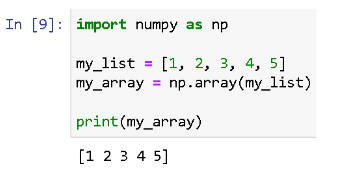Indexing and Slicing NumPy Arrays:

You can access individual elements of a NumPy array using indexing. NumPy arrays are zero-indexed, which means that the first element has an index of 0.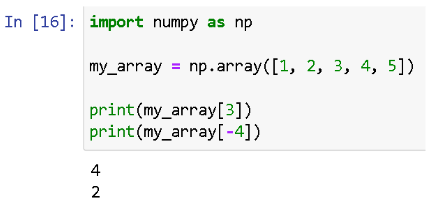You can also access a subset of elements from a NumPy array using slicing. Slicing allows you to extract a portion of an array based on a specified range of indices.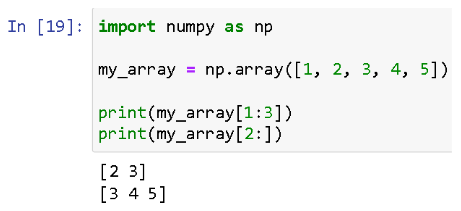Speeding up Your Code with NumPy: Vectorization and Performance Optimization

Vectorization is the process of performing operations on entire arrays or matrices at once, instead of using for loops to perform the same operations on individual elements. This can significantly improve the performance of your code since NumPy is optimised for these types of operations.

For example, suppose we have two 1D arrays a and b with 100 elements each, and we want to compute the dot product of the arrays. We could do this using a for loop, like so: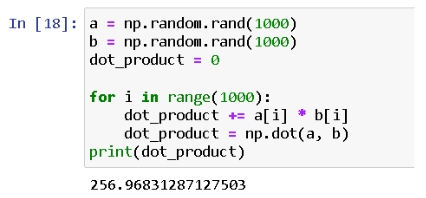This code is much simpler, and it is also much faster. In general, it is a good idea to use vectorization whenever possible, since it can simplify your code and improve performance.

Use NumPy Functions

NumPy provides a wide range of functions for performing mathematical operations on arrays and matrices. These functions are often highly optimised for performance, and they can be much faster than writing your own code using for loops.

For example, suppose we have a 2D array with 1000 rows and 100 columns, and we want to compute the mean of each column. We could do this using a for loop, like so: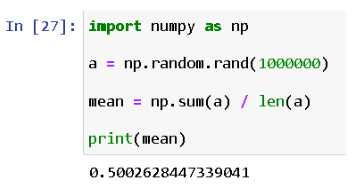This code is much simpler, and it is also much faster. In general, it is a good idea to use NumPy functions whenever possible, since they are often highly optimised for performance.

Arithmetic operations in NumPy

Maths Operations:

NumPy provides many built-in functions for performing maths operations on arrays.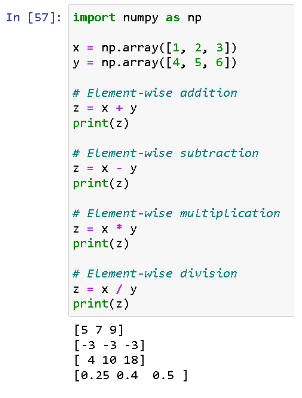Statistics Operations:

NumPy provides many built-in functions for performing statistics operations on arrays.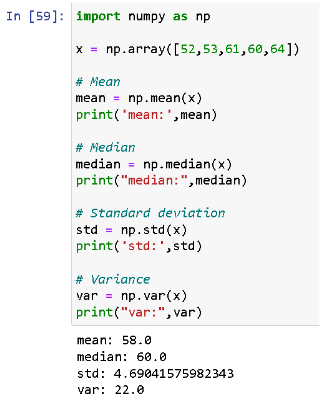Linear Algebra Operations:

NumPy provides many built-in functions for performing linear algebra operations on matrices.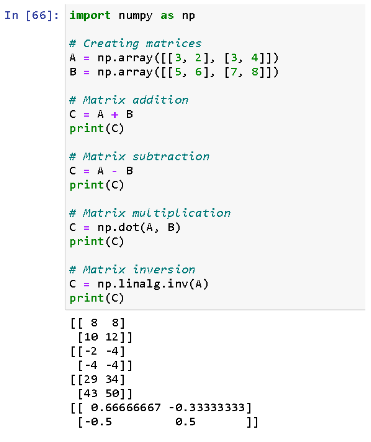Broadcasting is the ability of NumPy to perform arithmetic operations on arrays with different shapes and sizes, by stretching or duplicating the smaller array to match the shape of the larger array. Broadcasting is possible if the smaller array can be replicated along one or more axes to match the shape of the larger array.

For example, consider the following two arrays:

Array a has shape (2, 2) and the array b has shape (2,). To add these two arrays, we can use broadcasting: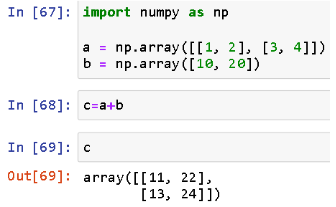In this example, the smaller array b was broadcast along the second axis to match the shape of the larger array a. The resulting array c has the same shape as a.

Broadcasting is a powerful feature of NumPy that allows us to perform arithmetic operations on arrays of different shapes and sizes. It is particularly useful when we want to avoid unnecessary copying of data, and when we want to write code that is more concise and readable.

#### NumPy Array vs. Python Lists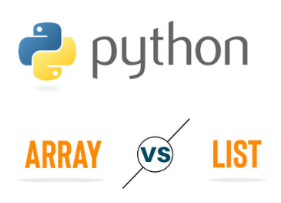When it comes to numerical computing in Python, you have two primary options: NumPy arrays or Python lists. While both have their benefits and drawbacks, NumPy arrays are generally considered to be the better option for numerical computing tasks.

#### Python Lists / NumPy Arrays

Python lists are a built-in data type in Python that allow you to store and manipulate collections of data. While they are easy to use and versatile, they are not ideal for numerical computing tasks.

NumPy is a powerful library in Python that provides a high-performance multidimensional array object, along with a suite of functions for performing mathematical operations on arrays.

#### Which is Better for Numerical Computing?

While both NumPy arrays and Python lists have their benefits and drawbacks, NumPy arrays are generally considered to be the better option for numerical computing tasks. With its statically typed arrays and suite of mathematical functions, NumPy provides a powerful tool for working with large datasets and performing complex mathematical operations.

#### Data Visualization with NumPy and Matplotlib.NumPy and Matplotlib, which is a powerful combination for data visualisation in Python. Scatter plots are useful for visualising the relationship between two variables, and in this case, we are using randomly generated data to demonstrate how to create a scatter plot with NumPy and Matplotlib.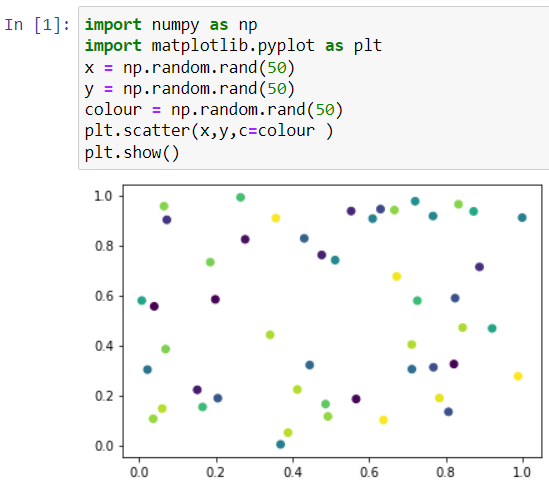#### Conclusion

NumPy is a powerful library for numerical computing in Python. It provides efficient array operations, mathematical functions, and tools for data manipulation and analysis. By utilizing NumPy, developers can perform complex computations with ease, handle multidimensional data structures effectively, and enhance the performance of their numerical tasks. Its integration with other libraries like Matplotlib further extends its capabilities for data visualization. NumPy is an essential tool for anyone working with numerical data in Python, offering simplicity, efficiency, and versatility.